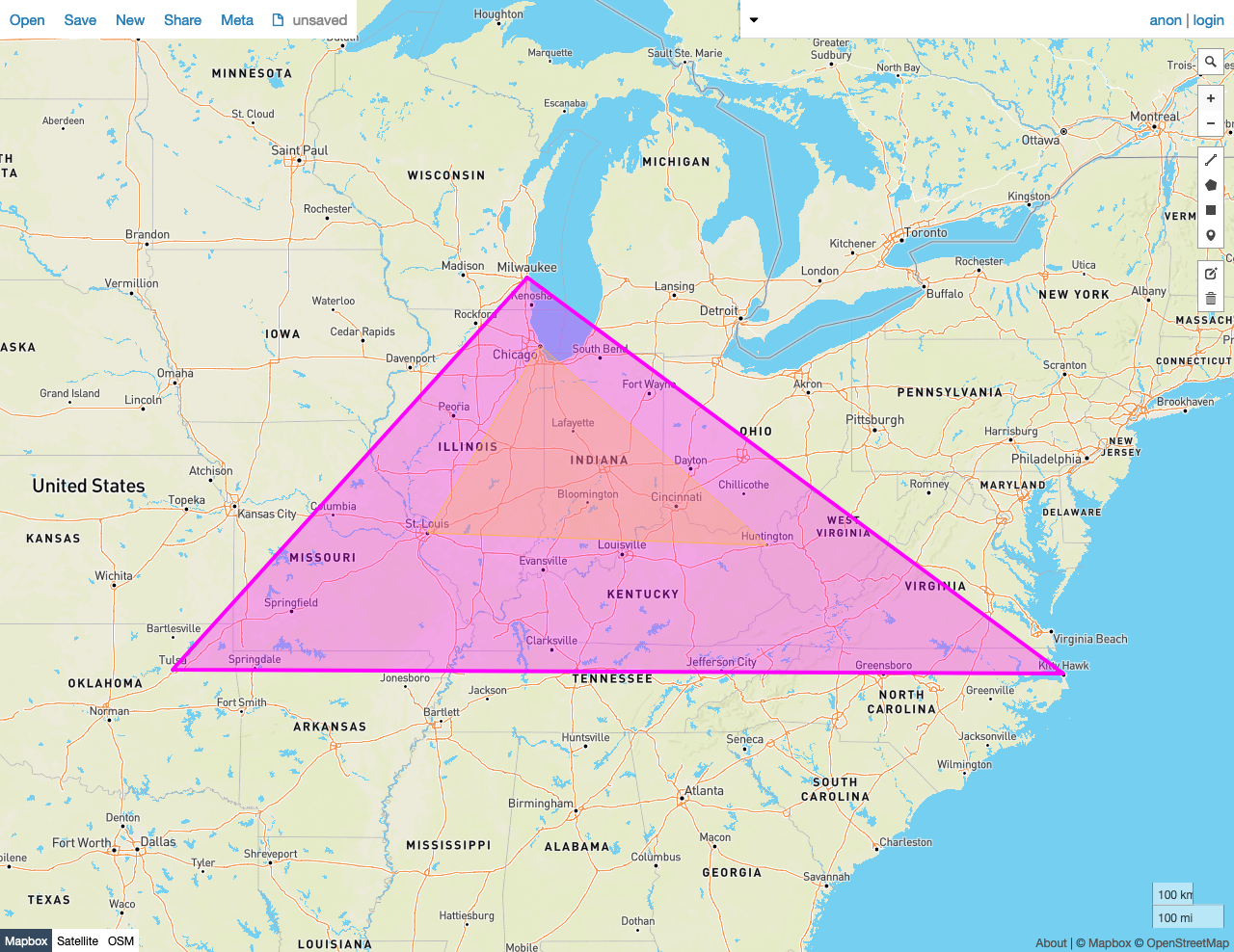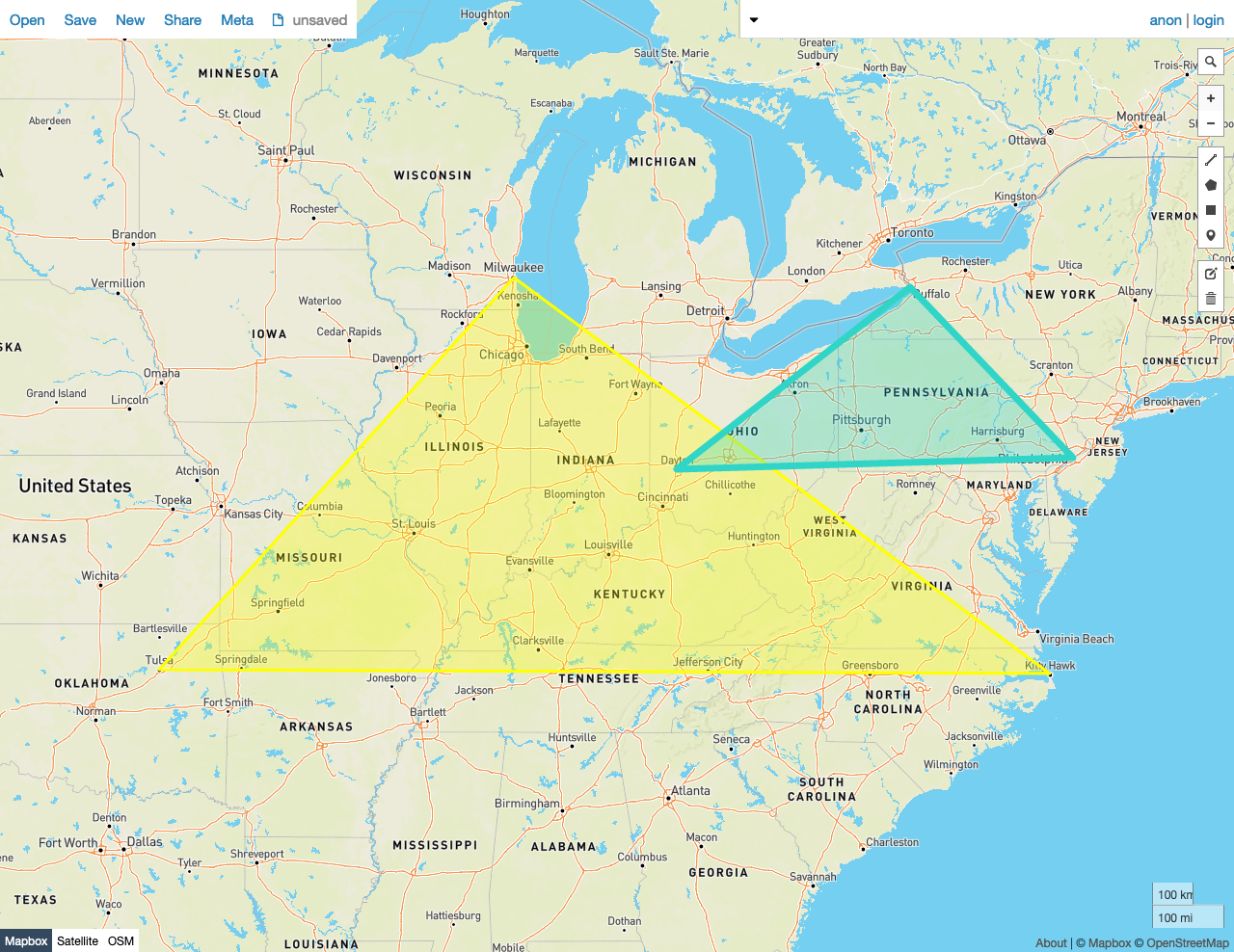Given two shapes A and B, the predicate function `ST_CoveredBy(A, B)` returns `true` if no point in A lies outside of shape B. Otherwise, it returns `false`.

In other words, shape B must completely cover every point in A.

`ST_CoveredBy` works on the following data types:

Note:

`ST_CoveredBy` will attempt to use any available spatial index to speed up its operation. Use the prefixed variant `_ST_CoveredBy` if you do not want any spatial indexes to be used.

Note:

This function is the inverse of `ST_Covers`.

## Examples

Note:

The screenshots in these examples were generated using geojson.io, but they are designed to showcase the shapes, not the map. Representing `GEOMETRY` data in GeoJSON can lead to unexpected results if using geometries with SRIDs other than 4326 (as shown below).

### True

In this example, `ST_CoveredBy` returns `true` because:

• No Point in the smaller Polygon A lies outside of the larger Polygon B.
``````SELECT ST_CoveredBy(st_geomfromtext('SRID=4326;POLYGON((-87.623177 41.881832, -90.199402 38.627003, -82.446732 38.413651, -87.623177 41.881832))'), st_geomfromtext('SRID=4326;POLYGON((-87.906471 43.038902, -95.992775 36.153980, -75.704722 36.076944, -87.906471 43.038902))'));
``````
``````  st_coveredby
----------------
true
(1 row)
``````### False

In this example, `ST_CoveredBy` returns `false` because:

• Many Points in the smaller Polygon A lie outside of the larger Polygon B.
``````SELECT ST_CoveredBy(st_geomfromtext('SRID=4326;POLYGON((-87.906471 43.038902, -95.992775 36.153980, -75.704722 36.076944, -87.906471 43.038902))'), st_geomfromtext('SRID=4326;POLYGON((-84.191605 39.758949, -75.165222 39.952583, -78.878738 42.880230, -84.191605 39.758949))'));
``````
``````  st_coveredby
----------------
false
(1 row)
``````